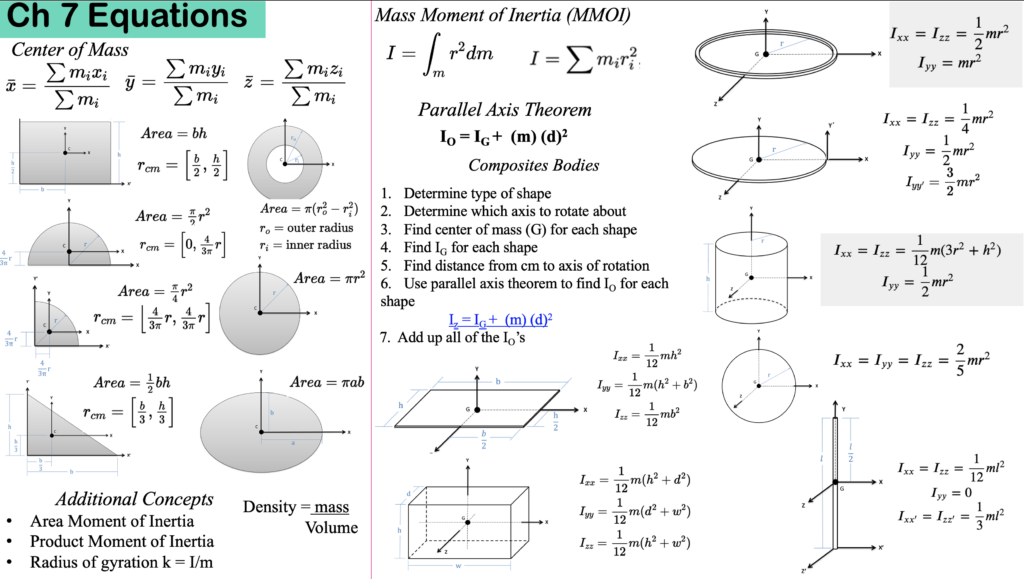# Chapter 7: Inertia

This chapter is a major preparation for objects that rotate during dynamics class. We will cover cg, inertia, composite shapes, and rotating about axes other than the centre of mass (cm) using the parallel axis theorem. Here are the sections in this Chapter:

Here are the important equations for this chapter: